# Search

About 563 Search Results Matching Grades matching 3rd Grade, Page 2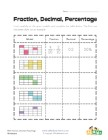## Fractions, Decimals and Percentages Worksheet 3

Use the model to figure out the fraction, decimal ...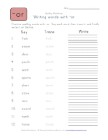## Say, Trace and Write -or Words Worksheet

Say, trace and then write each of the -or words in...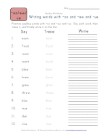## Say, Trace and Write -oo, -ew and -ue Words Worksheet

Say, trace and then write each of the -oo, -ew and...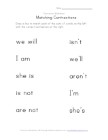## Matching Contractions

Draw a line to connect the contractions on one sid...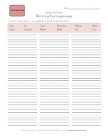## Writing Homophones Worksheet

Practice spelling by writing each of the homophone...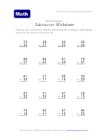## Subtraction Without Borrowing Worksheet 6

20 subtraction problems without borrowing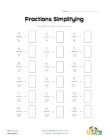## Fractions Simplifying Worksheet

Practice simplifying fractions with this worksheet...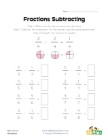## Simple Fraction Subtraction Worksheet 1

This fractions worksheet has 8 simple fraction sub...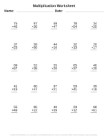## Multiplication Worksheet Generator

Generate printable multiplication worksheets using...